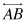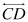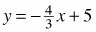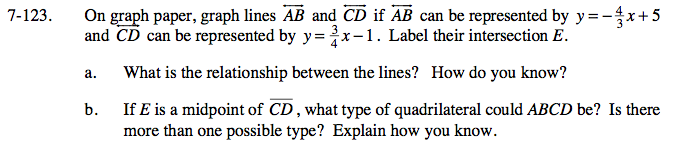### Home > GC > Chapter 7 > Lesson 7.3.3 > Problem7-123

7-123.
1. On graph paper, graph linesandifcan be represented byandcan be represented by y =− 1. Label their intersection E. Homework Help ✎

1. What is the relationship between the lines? How do you know?

2. If E is a midpoint of , what type of quadrilateral could ABCD be? Is there more than one possible type? Explain how you know.Examine each line's slope to determine the relationship between the two lines.

If E is a midpoint of CD, their diagonals would be perpendicular bisectors.